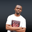Related Tags

php
strings
communitycreator

# How to print a formatted string in PHPNDUKWE CHIDERA K.

### Overview

A string is a data type that could be alphanumeric, numeric or alphabetical, but is enclosed in a single or double quote. Strings are displayed on the screen using regular output commands in PHP.

A formatted string is an interpolated string literal where placeholders are used in place of an actual value and are replaced during the evaluation of such strings with the value. It contains values that will be converted to the indicated type during evaluation.

Below is a sample formatted string.

### Return value

This function has a return value of the outputted string’s length.

### Code

<?php

$int = 4782;$anotherint = -4782;
$char = 57; // The ASCII Character 57 is 9 // Note: The format value "%%" returns a percent sign // Binary number printf("%%b = %b <br>",$int);
// The ASCII Character
printf("%%c = %c  ASCII Character converter<br>",$char); // Signed decimal number printf("%%d = %d is a Signed decimal number<br>",$anotherInt);
// Scientific notation (uppercase)
printf("%%E = %E is the Scientific notation of 4782 <br>",$int); // Unsigned decimal number (positive) printf("%%u = %u <br>",$int);
// Unsigned decimal number (negative)
printf("%%u = %u <br>",$anotherint); // Floating-point number (local settings aware) printf("%%f = %f <br>",$int);
// A string with converters inside it.
printf("These %1\$.2f are formatted strings %u examples. <br>",$int,$anotherint); ?> ### Explanation In the code above, different formatting styles were tried to show how they work. Take a close look at the inline comments to see what happened on each code line. Meanwhile, on the last code line in the snippet, the \$.2f format was used to set the floating point number to just two decimal places. In this format, \\$.2f, the slash was used to escape the ＄ dollar sign, which indicates that whatever is after it is an extra formatting option.

RELATED TAGS

php
strings
communitycreator

CONTRIBUTORNDUKWE CHIDERA K.
RELATED COURSES

View all Courses

Keep Exploring

Learn in-demand tech skills in half the time